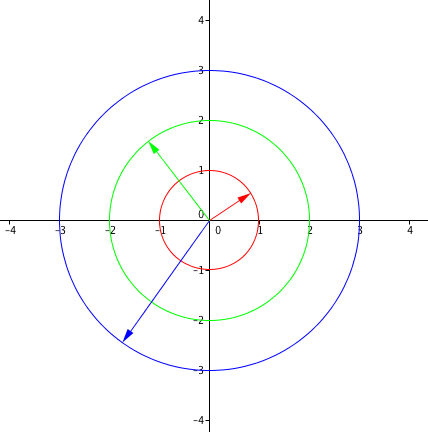# Absolute Value of Complex NumbersComplex numbers are in the form of $a+bi$, Where $i$ is the Imaginary Unit, defined as the square root of minus 1.

Absolute Value is often viewed as the "distance" a number is away from 0, the origin. In the domain of Real Numbers, This is just the positive of that number, so the absolute value of -5 will be 5 and the absolute value of 5 will also be 5.

When it comes to the absolute value of Complex Numbers, we have to consider the Complex Plane. The Complex Plane is similar to the xy-Coordinate Plane, only instead of the x and y axis you have the real and imaginary axis. The Number $3+4i$ will have coordinates (3,4) on the complex plane.

How will we find the distance a number is from 0 on the complex plane? We can use the Pythagorean Theorem! The Absolute Value of a complex number is defined as:

$|a+bi| = \sqrt{a^2 +b^2}$

For Example, the Absolute Value of the complex number $3+4i$ is equal to:

$|3+4i| = \sqrt{3^2 +4^2} = 5$Note by Yan Yau Cheng
6 years, 9 months ago

This discussion board is a place to discuss our Daily Challenges and the math and science related to those challenges. Explanations are more than just a solution — they should explain the steps and thinking strategies that you used to obtain the solution. Comments should further the discussion of math and science.

When posting on Brilliant:

• Use the emojis to react to an explanation, whether you're congratulating a job well done , or just really confused .
• Ask specific questions about the challenge or the steps in somebody's explanation. Well-posed questions can add a lot to the discussion, but posting "I don't understand!" doesn't help anyone.
• Try to contribute something new to the discussion, whether it is an extension, generalization or other idea related to the challenge.

MarkdownAppears as
*italics* or _italics_ italics
**bold** or __bold__ bold
- bulleted- list
• bulleted
• list
1. numbered2. list
1. numbered
2. list
Note: you must add a full line of space before and after lists for them to show up correctly
paragraph 1paragraph 2

paragraph 1

paragraph 2

[example link](https://brilliant.org)example link
> This is a quote
This is a quote
    # I indented these lines
# 4 spaces, and now they show
# up as a code block.

print "hello world"
# I indented these lines
# 4 spaces, and now they show
# up as a code block.

print "hello world"
MathAppears as
Remember to wrap math in $$ ... $$ or $ ... $ to ensure proper formatting.
2 \times 3 $2 \times 3$
2^{34} $2^{34}$
a_{i-1} $a_{i-1}$
\frac{2}{3} $\frac{2}{3}$
\sqrt{2} $\sqrt{2}$
\sum_{i=1}^3 $\sum_{i=1}^3$
\sin \theta $\sin \theta$
\boxed{123} $\boxed{123}$

Sort by:

Also, note that the "absolute value" of a complex number is also called the "magnitude." I myself tend to use magnitude for non-real numbers, and absolute value for real numbers.

- 6 years, 9 months ago

Problem: What is the absolute value of the product of two complex numbers? Use specific examples to aid in your findings. Can you generalize your statement in any way?

- 6 years, 9 months ago

@Yan Yau Cheng Can you add this to the Wiki page of Complex Numbers - Absolute Values? Thanks!

Staff - 5 years, 11 months ago